Download PresentationIntegers!!!

# Integers!!! - PowerPoint PPT Presentation

Integers!!! INTEGERS!!!! Numbers can be both positive and negative. When working with negative numbers there are rules that must be followed. Think of some ways that you use negative numbers???? Visualisation Think of a greenhouse.I am the owner, or an agent authorized to act on behalf of the owner, of the copyrighted work described.
Download Presentation## Integers!!!

An Image/Link below is provided (as is) to download presentation

Download Policy: Content on the Website is provided to you AS IS for your information and personal use and may not be sold / licensed / shared on other websites without getting consent from its author.While downloading, if for some reason you are not able to download a presentation, the publisher may have deleted the file from their server.

- - - - - - - - - - - - - - - - - - - - - - - - - - E N D - - - - - - - - - - - - - - - - - - - - - - - - - -
Presentation Transcript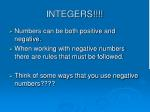INTEGERS!!!!
• Numbers can be both positive and negative.
• When working with negative numbers there are rules that must be followed.
• Think of some ways that you use negative numbers????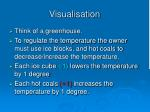Visualisation
• Think of a greenhouse.
• To regulate the temperature the owner must use ice blocks, and hot coals to decrease/increase the temperature.
• Each ice cube (-1) lowers the temperature by 1 degree
• Each hot coals (+1) increases the temperature by 1 degree.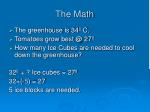The Math
• The greenhouse is 34º C.
• Tomatoes grow best @ 27º
• How many Ice Cubes are needed to cool down the greenhouse?

32º + ? Ice cubes = 27º

32+(-5) = 27

5 ice blocks are needed.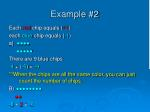Example #2

Each red chip equals (+1)

each blue chip equals (-1)

a) ●●●●

●●●●●

There are 9 blue chips

-4 + (-5)= -9

**When the chips are all the same color, you can just count the number of chips.

B) ●●●●●●●●

●●

-8 + 2 = -6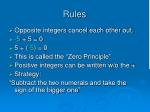Rules
• Opposite integers cancel each other out.
• -5 + 5 = 0
• 5 + (-5) = 0
• This is called the “Zero Principle”
• Positive integers can be written w/o the +
• Strategy:

“Subtract the two numerals and take the sign of the bigger one”Subtracting Integers
• 3 – (-2)

●●●

= ●●● ●● ●●

= ●●● ●● ●●

= ●●● ●●

Start with 3 Red. Add 2 blue and 2 red using the zero principle. Take away 2 blue to subtract -2. The integer chips represent the sum 3 + 2. The answer is 5. You can subtract an integer by adding its opposite.Method #2
• Instead of subtracting an integer, we may add the opposite!

A) -4 – (-2)

= -4 + 2

= -2

B) -3 – 5

= - 3 + (-5)

= -8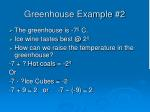Greenhouse Example #2
• The greenhouse is -7º C.
• Ice wine tastes best @ 2º
• How can we raise the temperature in the greenhouse?

-7 + ? Hot coals = -2º

Or

-7 - ?Ice Cubes = -2

-7 + 9 = 2 or -7 – (-9) = 2